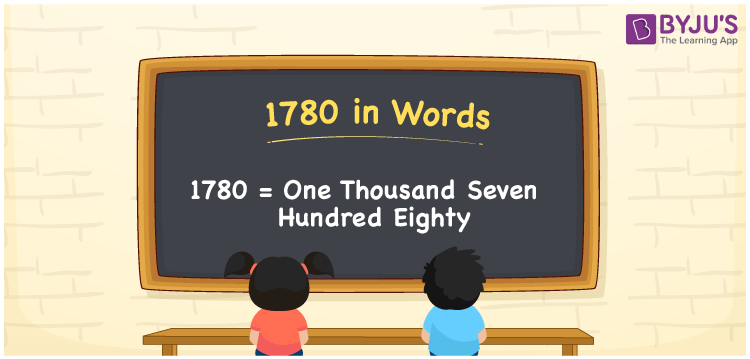# 1780 in words

1780 in words is written as One Thousand Seven Hundred and Eighty. 1780 represents the count or value. The article on Counting Numbers can give you an idea about count or counting. The number 1780 is used in expressions that relate to money, distance, length, year and others. Let us consider an example for 1780. “I could only save One Thousand Seven Hundred and Eighty rupees this month .” Another example, “The number of people residing in a posh society is One Thousand Seven Hundred and Eighty.”

 1780 in words One Thousand Seven Hundred and Eighty One Thousand Seven Hundred and Eighty in Numbers 1780

## 1780 in English Words## How to Write 1780 in Words?

We can convert 1780 to words using a place value chart. The number 1780 has 4 digits, so let’s make a chart that shows the place value up to 4 digits.

 Thousands Hundreds Tens Ones 1 7 8 0

Thus, we can write the expanded form as:

1 × Thousand + 7 × Hundred + 8 × Ten + 0 × One

= 1 × 1000 + 7 × 100 + 8 × 10 + 0 × 1

= 1780

= One Thousand Seven Hundred and Eighty.

1780 is the natural number that is succeeded by 1779 and preceded by 1781.

1780 in words – One Thousand Seven Hundred and Eighty.

Is 1780 an odd number? – No.

Is 1780 an even number? – Yes.

Is 1780 a perfect square number? – No.

Is 1780 a perfect cube number? – No.

Is 1780 a prime number? – No.

Is 1780 a composite number? – Yes.

## Solved Example

1. Write the number 1780 in expanded form

Solution: 1 × 1000 + 7 × 100 + 8 × 10 + 0 × 1

We can write 1780 = 1000 + 700 + 80 + 0

= 1 × 1000 + 7 × 100 + 8 × 10 + 0 × 1

## Frequently Asked Questions on 1780 in words

Q1

### How to write 1780 in words?

1780 in words is written as One Thousand Seven Hundred and Eighty.
Q2

### State if True or False. 1780 is divisible by 2?

True. 1780 is divisible by 2.
Q3

### Is 1780 a perfect square number?

No. 1780 is not a perfect square number.